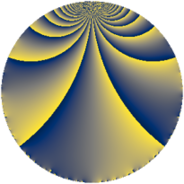# Properties

 Label 7728.2.a.boLevel $7728$ Weight $2$ Character orbit 7728.a Self dual yes Analytic conductor $61.708$ Analytic rank $0$ Dimension $2$ CM no Inner twists $1$

# Related objects

## Newspace parameters

 Level: $$N$$ $$=$$ $$7728 = 2^{4} \cdot 3 \cdot 7 \cdot 23$$ Weight: $$k$$ $$=$$ $$2$$ Character orbit: $$[\chi]$$ $$=$$ 7728.a (trivial)

## Newform invariants

 Self dual: yes Analytic conductor: $$61.7083906820$$ Analytic rank: $$0$$ Dimension: $$2$$ Coefficient field: $$\Q(\sqrt{5})$$ Defining polynomial: $$x^{2} - x - 1$$ Coefficient ring: $$\Z[a_1, \ldots, a_{5}]$$ Coefficient ring index: $$1$$ Twist minimal: no (minimal twist has level 1932) Fricke sign: $$-1$$ Sato-Tate group: $\mathrm{SU}(2)$

## $q$-expansion

Coefficients of the $$q$$-expansion are expressed in terms of $$\beta = \frac{1}{2}(1 + \sqrt{5})$$. We also show the integral $$q$$-expansion of the trace form.

 $$f(q)$$ $$=$$ $$q + q^{3} + \beta q^{5} + q^{7} + q^{9} +O(q^{10})$$ $$q + q^{3} + \beta q^{5} + q^{7} + q^{9} + ( 1 + 2 \beta ) q^{11} + ( 1 + \beta ) q^{13} + \beta q^{15} + ( -1 + 2 \beta ) q^{17} + ( -1 + 4 \beta ) q^{19} + q^{21} - q^{23} + ( -4 + \beta ) q^{25} + q^{27} + ( 3 - 4 \beta ) q^{29} + ( 3 + 2 \beta ) q^{31} + ( 1 + 2 \beta ) q^{33} + \beta q^{35} + ( -3 + 4 \beta ) q^{37} + ( 1 + \beta ) q^{39} + ( 5 - 4 \beta ) q^{41} + ( 7 - 9 \beta ) q^{43} + \beta q^{45} + ( 2 + 2 \beta ) q^{47} + q^{49} + ( -1 + 2 \beta ) q^{51} + ( -4 + 5 \beta ) q^{53} + ( 2 + 3 \beta ) q^{55} + ( -1 + 4 \beta ) q^{57} + ( 4 + \beta ) q^{59} + ( 1 - 7 \beta ) q^{61} + q^{63} + ( 1 + 2 \beta ) q^{65} + ( 4 + \beta ) q^{67} - q^{69} + ( 9 - 3 \beta ) q^{71} + ( -3 - 8 \beta ) q^{73} + ( -4 + \beta ) q^{75} + ( 1 + 2 \beta ) q^{77} + ( 7 - 2 \beta ) q^{79} + q^{81} + ( 3 - 8 \beta ) q^{83} + ( 2 + \beta ) q^{85} + ( 3 - 4 \beta ) q^{87} + ( -1 + 3 \beta ) q^{89} + ( 1 + \beta ) q^{91} + ( 3 + 2 \beta ) q^{93} + ( 4 + 3 \beta ) q^{95} + ( 1 + 2 \beta ) q^{97} + ( 1 + 2 \beta ) q^{99} +O(q^{100})$$ $$\operatorname{Tr}(f)(q)$$ $$=$$ $$2q + 2q^{3} + q^{5} + 2q^{7} + 2q^{9} + O(q^{10})$$ $$2q + 2q^{3} + q^{5} + 2q^{7} + 2q^{9} + 4q^{11} + 3q^{13} + q^{15} + 2q^{19} + 2q^{21} - 2q^{23} - 7q^{25} + 2q^{27} + 2q^{29} + 8q^{31} + 4q^{33} + q^{35} - 2q^{37} + 3q^{39} + 6q^{41} + 5q^{43} + q^{45} + 6q^{47} + 2q^{49} - 3q^{53} + 7q^{55} + 2q^{57} + 9q^{59} - 5q^{61} + 2q^{63} + 4q^{65} + 9q^{67} - 2q^{69} + 15q^{71} - 14q^{73} - 7q^{75} + 4q^{77} + 12q^{79} + 2q^{81} - 2q^{83} + 5q^{85} + 2q^{87} + q^{89} + 3q^{91} + 8q^{93} + 11q^{95} + 4q^{97} + 4q^{99} + O(q^{100})$$

## Embeddings

For each embedding $$\iota_m$$ of the coefficient field, the values $$\iota_m(a_n)$$ are shown below.

For more information on an embedded modular form you can click on its label.

Label $$\iota_m(\nu)$$ $$a_{2}$$ $$a_{3}$$ $$a_{4}$$ $$a_{5}$$ $$a_{6}$$ $$a_{7}$$ $$a_{8}$$ $$a_{9}$$ $$a_{10}$$
1.1
 −0.618034 1.61803
0 1.00000 0 −0.618034 0 1.00000 0 1.00000 0
1.2 0 1.00000 0 1.61803 0 1.00000 0 1.00000 0
 $$n$$: e.g. 2-40 or 990-1000 Significant digits: Format: Complex embeddings Normalized embeddings Satake parameters Satake angles

## Atkin-Lehner signs

$$p$$ Sign
$$2$$ $$-1$$
$$3$$ $$-1$$
$$7$$ $$-1$$
$$23$$ $$1$$

## Inner twists

This newform does not admit any (nontrivial) inner twists.

## Twists

By twisting character orbit
Char Parity Ord Mult Type Twist Min Dim
1.a even 1 1 trivial 7728.2.a.bo 2
4.b odd 2 1 1932.2.a.d 2
12.b even 2 1 5796.2.a.i 2

By twisted newform orbit
Twist Min Dim Char Parity Ord Mult Type
1932.2.a.d 2 4.b odd 2 1
5796.2.a.i 2 12.b even 2 1
7728.2.a.bo 2 1.a even 1 1 trivial

## Hecke kernels

This newform subspace can be constructed as the intersection of the kernels of the following linear operators acting on $$S_{2}^{\mathrm{new}}(\Gamma_0(7728))$$:

 $$T_{5}^{2} - T_{5} - 1$$ $$T_{11}^{2} - 4 T_{11} - 1$$ $$T_{13}^{2} - 3 T_{13} + 1$$ $$T_{17}^{2} - 5$$

## Hecke characteristic polynomials

$p$ $F_p(T)$
$2$ $$T^{2}$$
$3$ $$( -1 + T )^{2}$$
$5$ $$-1 - T + T^{2}$$
$7$ $$( -1 + T )^{2}$$
$11$ $$-1 - 4 T + T^{2}$$
$13$ $$1 - 3 T + T^{2}$$
$17$ $$-5 + T^{2}$$
$19$ $$-19 - 2 T + T^{2}$$
$23$ $$( 1 + T )^{2}$$
$29$ $$-19 - 2 T + T^{2}$$
$31$ $$11 - 8 T + T^{2}$$
$37$ $$-19 + 2 T + T^{2}$$
$41$ $$-11 - 6 T + T^{2}$$
$43$ $$-95 - 5 T + T^{2}$$
$47$ $$4 - 6 T + T^{2}$$
$53$ $$-29 + 3 T + T^{2}$$
$59$ $$19 - 9 T + T^{2}$$
$61$ $$-55 + 5 T + T^{2}$$
$67$ $$19 - 9 T + T^{2}$$
$71$ $$45 - 15 T + T^{2}$$
$73$ $$-31 + 14 T + T^{2}$$
$79$ $$31 - 12 T + T^{2}$$
$83$ $$-79 + 2 T + T^{2}$$
$89$ $$-11 - T + T^{2}$$
$97$ $$-1 - 4 T + T^{2}$$